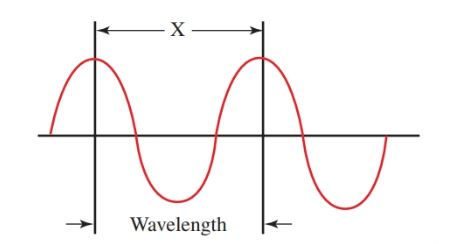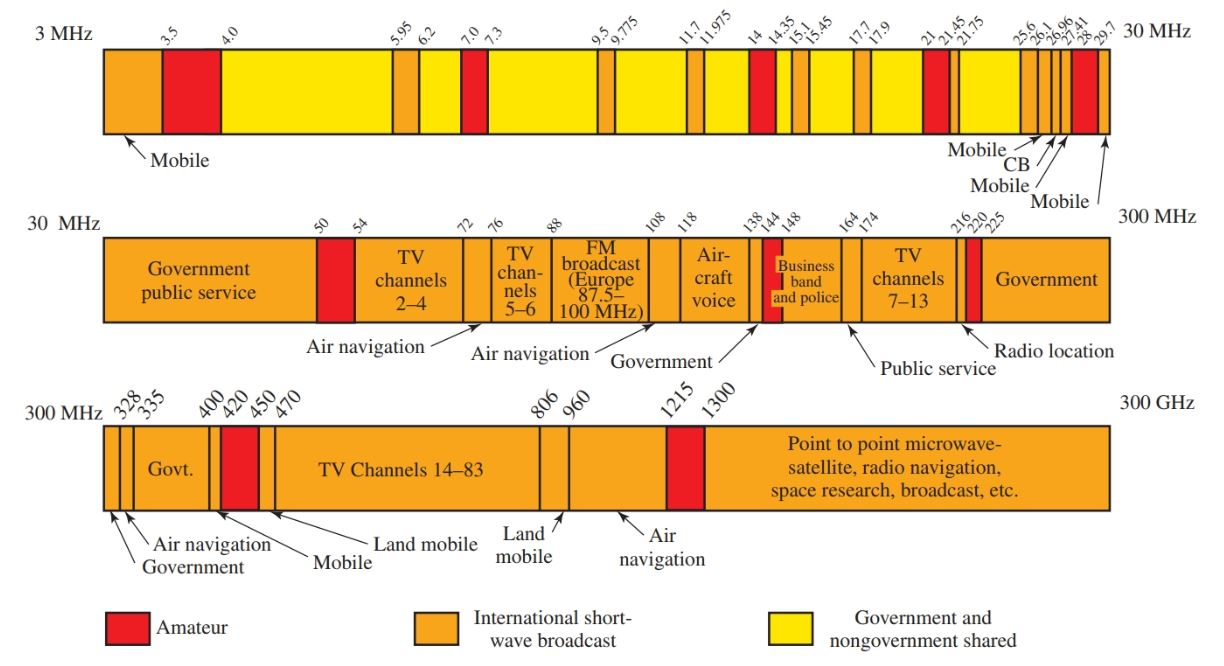# Radio Waves Frequency and Wavelength

Want create site? Find Free WordPress Themes and plugins.

Radio waves travel through space at a speed of light, which is 186,000 miles per second (300,000,000 meters per second). This is the velocity of a radio wave.

Refer to Figure 1. The first radio wave has a frequency of one cycle per second (1 Hz). Starting at point A, the wave will move 186,000 miles by the time it completes one cycle and reaches point B.

In the second wave, the frequency of the wave is 1000 Hz. The wave will move 186 miles by the time it completes one cycle and reaches point B. The second wave completes its one cycle in 1/1000th of a second.

In the third wave, the frequency is increased to 1,000,000 Hz or 1 megahertz. The wave will move only 0.186 miles by the time it completes one cycle and reaches point B.Figure 1. A comparison of the distances traveled in one cycle for 1 Hz, 1000 Hz, and 1,000,000 Hz waves.

A wave can be described not only by its frequency, then, but also by its length, Figure 2. The distance between the crests of the waves is the wavelength. As the frequency increases, the wavelength decreases. The Greek letter λ (lambda) stands for wavelength.Figure 2. Radio waves are identified by their length and their frequency. X equals one wave.

The length of a radio wave is important and must be taken into consideration when designing communication systems. The mathematical relationship of frequency and wavelength is:

$\lambda =\frac{V}{f}$

Where is the wavelength given in miles, V is the velocity of the wave given in miles per second, and f is the frequency in hertz. Since the waves travel at the speed of light, the formula can be written:

$\lambda =\frac{186,000\text{ }miles\text{ }per\text{ }second}{f}$

To solve for frequency, the equation is rearranged to read:

$f=\frac{186,000\text{ }miles\text{ }per\text{ }second}{\lambda }$

When using metric measurements, the formulas are written:

$\lambda =\frac{300,000,000\text{ }meters\text{ }per\text{ }second}{f}$

And,

$f=\frac{300,000,000\text{ }meters\text{ }per\text{ }second}{\lambda }$

Where is the wavelength given in meters and f is the frequency in hertz.

## Radio Wave Wavelength Calculation Example

An amateur radio broadcaster transmits on a frequency of 3.9 MHz. What is the wavelength of the radiated waves?

$\lambda =\frac{186,000\text{ }miles\text{ }per\text{ }second}{3.9MHz}$

First, 3.9 MHz is converted to 3,900,000 Hz.

$\lambda =\frac{186,000\text{ }miles\text{ }per\text{ }second}{3,900,000Hz}$

Observe how the math is simplified by using powers of ten.

$\lambda =\frac{1.86\times {{10}^{5}}}{3.9\times {{10}^{6}}}\cong 0.05miles$

## Radio Wave Frequency Calculation Example

What is the frequency of a transmitter operating on 40 meters?

$f=\frac{3\times {{10}^{8}}\text{ }meters\text{ }per\text{ }second}{4\times 10\text{ }meters}=0.75\times {{10}^{7}}=7.5MHz$

A more useful form of this formula is available. Since the foot is the common unit of measure and radio frequencies are usually in megahertz:

$\lambda =\frac{984}{f}$

Where is wavelength measured in feet and f is frequency measured in megahertz.

Memorize these formulas. They are used a great deal in the design of special frequency antennas. The antenna absorbs most of the radio wave when it matches the length of the radio wave.

## Frequency Spectrum

Electromagnetic waves are produced at many different frequencies. Since the frequencies of radio transmitters could interfere with each other, a system of regulating the use of transmitters had to be developed. This system ensures an orderly use of the airwaves. See Figure 3. This system is known as a frequency spectrum.

This chart shows the frequency bands used by telecommunication areas such as radio, television, satellite transmission, aviation, radar, police, and many more.Figure 3. Frequency spectrum assignments. (Tektronix)

The use of frequencies is strictly controlled. This is because there are many users of these systems. And each user requires a frequency on which to broadcast.

Since there is only a limited number of frequencies available, the frequencies must be assigned to meet the needs of the public. At the same time, there must be a minimum of interference between the services. The Federal Communications Commission (FCC) controls the frequency assignment in the United States.

Waves are also grouped according to frequency, Figure 4. Note the location of some services.Figure 4. Study this chart of frequency ranges. Can you name one device that operates in each band?

AM broadcast stations use the MF range. Television uses the VHF and UHF ranges. A stereo receiver operates in the audio frequency range. Electricity for the home is 60 Hz. It operates at the audio frequency.

Did you find apk for android? You can find new Free Android Games and apps.